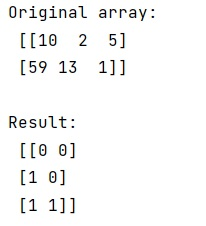# How to get indices of elements that are greater than a threshold in 2D NumPy array?

Learn, how to get indices of elements that are greater than a threshold in 2D NumPy array?
Submitted by Pranit Sharma, on March 02, 2023

NumPy is an abbreviated form of Numerical Python. It is used for different types of scientific operations in python. Numpy is a vast library in python which is used for almost every kind of scientific or mathematical operation. It is itself an array which is a collection of various methods and functions for processing the arrays.

## Getting the indices of elements that are greater than a threshold in 2D NumPy array

Suppose that we are given a 2D numpy array that contains some numerical values and we need to get the indices of those values which are greater than a threshold value (say 5).

For this purpose, we can use numpy.argwhere() to return the indices of all the entries in an array matching a boolean condition.
We need to pass a condition that (arr > 5) inside argwhere() as a parameter and it will return the index of that value where the result is True.

Let us understand with the help of an example,

## Python code to get indices of elements that are greater than a threshold in 2D NumPy array

```# Import numpy
import numpy as np

# Creating a numpy array
arr = np.array([[10,2,5],[59,13,1]])

# Display original array
print("Original array:\n",arr,"\n")

# Returning indices of values
# greater than 5
res = np.argwhere(arr>5)

# Display result
print("Result:\n",res,"\n")
```

Output:Languages: » C » C++ » C++ STL » Java » Data Structure » C#.Net » Android » Kotlin » SQL
Web Technologies: » PHP » Python » JavaScript » CSS » Ajax » Node.js » Web programming/HTML
Solved programs: » C » C++ » DS » Java » C#
Aptitude que. & ans.: » C » C++ » Java » DBMS
Interview que. & ans.: » C » Embedded C » Java » SEO » HR
CS Subjects: » CS Basics » O.S. » Networks » DBMS » Embedded Systems » Cloud Computing
» Machine learning » CS Organizations » Linux » DOS
More: » Articles » Puzzles » News/Updates